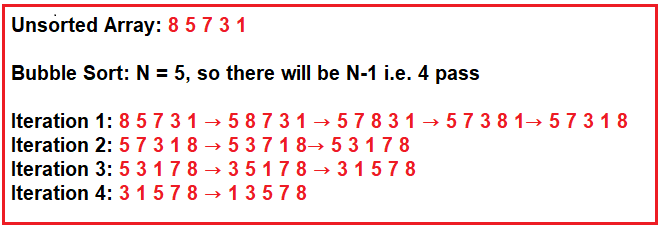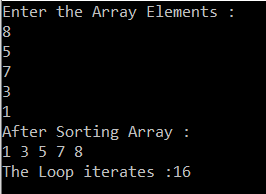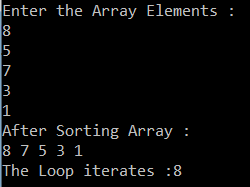# Bubble Sort in C#

## Bubble Sort in C# with Examples

In this article, I am going to discuss the Bubble Sort in C# with Examples. The Bubble sort is a sorting algorithm and used by many developers in real-time applications. You can use this algorithm with any type of collection such as an array, string, numbers, or characters.

##### The Bubble Sort Algorithm:

The Bubble Sort Algorithm works on the concept of iterating through the array from the first index to the last index and comparing with the adjacent elements and then swapping the elements if they appear in the wrong order i.e. if the next element is smaller than the current element, they are swapped.

##### Pictorial Representation of Bubble Sort:

In order to sort an array, there will be n-1 passes where n is the number of elements in the array. For better understanding please have a look at the following diagram. Let us take an input array such as 8 5 7 3 1.As you can see in the above image we have an integer array with 5 elements such as 8 5 7 3 1.

###### Iteration1:

The bubble sort starts with the first two elements i.e. 8 and 5. As 5 is smaller than 8, so swap both of them. Now the list is 5 8 7 3 1. Again 7 is less than 8, so swap them which result as 5 7 8 3 1. Now, 3 is less than 8, so swap them which results in a sequence like 5 7 3 8 1. Finally 1 is less than 8, so swap them which concludes the iteration1 as 5 7 3 1 and 8.

###### Iteration2:

For iteration2 the sequence is 5 7 3 1 and 8. Here, the first 2 elements are 5 and 7. 7 is greater than 5, so no need to swap them. The 7 is compared with 3 and the 3 is less than 7, so swap them which results in the sequences as 5 3 7 1 8. Then 1 is less than 7 so swap them which results in a sequence like 5 3 1 7 8.

###### Iteration3:

For iteration3, the sequence will be 5 3 1 7 8. Here, the first two elements are 5 and 3. 3 is less than 5, so swap them which results in the sequences as 3 5 1 7, and 8. Again 1 is less than 5, so swap them which concludes iteration3 with the sequences as 3 1 5 7 and 8.

###### Iteration4:

This is the last iteration. This iteration starts with the sequence as 3 1 5 7 and 8. Here the first two elements are 3 and 1. 1 is less than 3, so swap them which results in the elements in the sorted order as 1 3 5 7 and 8.

##### Program to implement bubble sort in C#:
```using System;
namespace LogicalPrograms
{
class Program
{
static void Main(string[] args)
{
int count = 0;
int[] intArray = new int;
Console.WriteLine("Enter the Array Elements : ");
for (int i = 0; i < intArray.Length; i++)
{
}

//Sorting the array
for (int j = 0; j <= intArray.Length - 2; j++)
{
//intArray.Length - 2
for (int i = 0; i <= intArray.Length - 2; i++)
{
count = count + 1;
if (intArray[i] > intArray[i + 1])
{
int temp = intArray[i + 1];
intArray[i + 1] = intArray[i];
intArray[i] = temp;
}
}
}

Console.WriteLine("After Sorting Array :");
foreach (int item in intArray)
{
Console.Write(item + " ");
}
Console.WriteLine();
Console.WriteLine("The Loop iterates :" + count);
}
}
}```
###### Output:As you can see the number of iterates is 16. You can improve the performance of bubble sort by using a bool flag.

##### Performance Improvement in Bubble sort:

In the following example, we are using a flag variable.

```using System;
namespace LogicalPrograms
{
class Program
{
static void Main(string[] args)
{
int count = 0;
int[] intArray = new int;
Console.WriteLine("Enter the Array Elements : ");
for (int i = 0; i < intArray.Length; i++)
{
}

bool flag = true;

for (int i = 1; (i <= (intArray.Length - 1)) && flag; i++)
{
flag = false;
for (int j = 0; j < (intArray.Length - 1); j++)
{
count = count + 1;
if (intArray[j + 1] > intArray[j])
{
int temp = intArray[j];
intArray[j] = intArray[j + 1];
intArray[j + 1] = temp;
flag = true;
}
}
}

Console.WriteLine("After Sorting Array :");
foreach (int item in intArray)
{
Console.Write(item + " ");
}
Console.WriteLine();
Console.WriteLine("The Loop iterates :" + count);
}
}
}
```
###### Output:##### Time Complexity of Bubble Sort:

In bubble sort, as we are iterating through the entire array for each element, the average and the worst-case complexity of bubble sort is O(n²).

##### Algorithm for Bubble Sort:

Procedure BubbleSort(DATA: list of sortable items)
N= DATA.Length

1. Set Flag: = True
2. Repeat Steps from 3 to 5 for I = 1 to N-1 while Flag == true
3. Set Flag:= False
4. Set J:=0. [Initialize pass pointer J]
5. Repeat while J<N-1 [Executes pass]
(a) If DATA[J+1]>DATA[J], then:
Swap DATA[J] and DATA[J+1]
Set Flag:= True
[End of If structure]

(b) Set J:=J+1
[End of inner loop]
[End of step 1 outer loop]

6. Exit

In the next article, I am going to discuss the Merge Sort Algorithm In C# with examples. Here, in this article, I try to explain the Bubble Sort in C# with Examples. I hope now you understood how bubble sort works in C# and I also hope you enjoy this article.